Submitted Successfully!
Thank you for your contribution! You can also upload a video entry or images related to this topic.
Ver. Summary Created by Modification Content Size Created at Operation
1 handwiki -- 1836 2022-11-28 01:32:33 |
2 format corrected. + 87 word(s) 1923 2022-11-30 12:10:30 |

Do you have a full video?

# Confirm

Are you sure to Delete?
Cite
Zheng, H. Binary Adder. Encyclopedia. Available online: https://encyclopedia.pub/entry/37135 (accessed on 30 November 2023).
Zheng H. Binary Adder. Encyclopedia. Available at: https://encyclopedia.pub/entry/37135. Accessed November 30, 2023.
Zheng, Handwiki. "Binary Adder" Encyclopedia, https://encyclopedia.pub/entry/37135 (accessed November 30, 2023).
Zheng, H.(2022, November 29). Binary Adder. In Encyclopedia. https://encyclopedia.pub/entry/37135
Zheng, Handwiki. "Binary Adder." Encyclopedia. Web. 29 November, 2022.

An adder, or summer, is a digital circuit that performs addition of numbers. In many computers and other kinds of processors adders are used in the arithmetic logic units (ALUs). They are also used in other parts of the processor, where they are used to calculate addresses, table indices, increment and decrement operators and similar operations. Although adders can be constructed for many number representations, such as binary-coded decimal or excess-3, the most common adders operate on binary numbers. In cases where two's complement or ones' complement is being used to represent negative numbers, it is trivial to modify an adder into an adder–subtractor. Other signed number representations require more logic around the basic adder.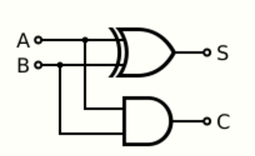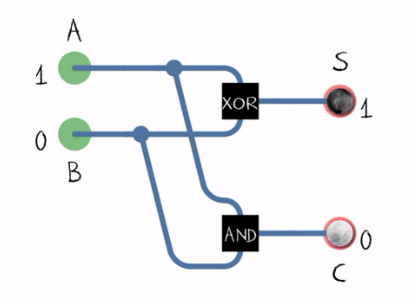Inputs Outputs
A B C S
0 0 0 0
0 1 0 1
1 0 0 1
1 1 1 0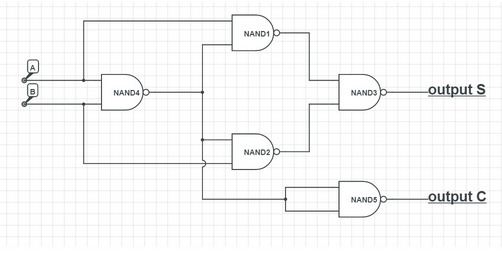Half adder using NAND gates only. https://handwiki.org/wiki/index.php?curid=1533413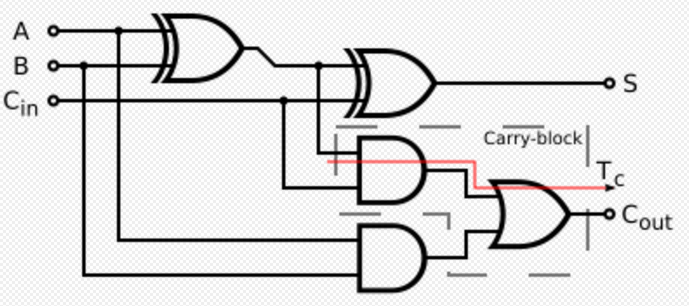Logic diagram for a full adder. https://handwiki.org/wiki/index.php?curid=2065042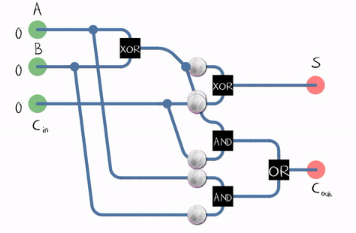Full adder in action. A full adder gives the number of 1s in the input in binary representation. https://handwiki.org/wiki/index.php?curid=1519448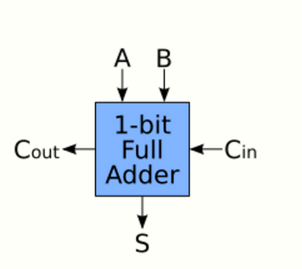Schematic symbol for a 1-bit full adder with Cin and Cout drawn on sides of block to emphasize their use in a multi-bit adder. https://handwiki.org/wiki/index.php?curid=2051549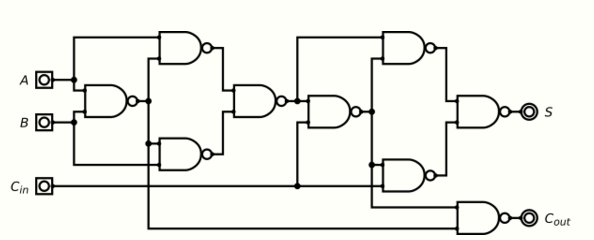Full adder built up from nine NAND gates. https://handwiki.org/wiki/index.php?curid=2065686

A full adder adds binary numbers and accounts for values carried in as well as out. A one-bit full-adder adds three one-bit numbers, often written as A, B, and Cin; A and B are the operands, and Cin is a bit carried in from the previous less-significant stage. The full adder is usually a component in a cascade of adders, which add 8, 16, 32, etc. bit binary numbers. The circuit produces a two-bit output. Output carry and sum typically represented by the signals Cout and S, where the sum equals 2Cout + S.

A full adder can be implemented in many different ways such as with a custom transistor-level circuit or composed of other gates. One example implementation is with S = ABCin and Cout = (AB) + (Cin ⋅ (AB)).

In this implementation, the final OR gate before the carry-out output may be replaced by an XOR gate without altering the resulting logic. This is beacause when A and B are both 1, the term (AB) is always 0, and hence (Cin ⋅ (AB)) can only be 0. Thus, the inputs to the final OR gate can never be both 1's (this is the only combination for which the OR and XOR outputs differ).

Using only two types of gates is convenient if the circuit is being implemented using simple integrated circuit chips which contain only one gate type per chip.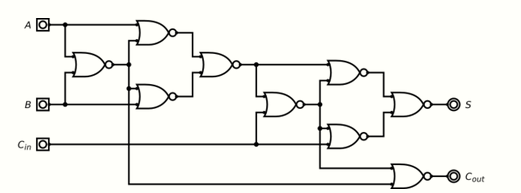A full adder can also be constructed from two half adders by connecting A and B to the input of one half adder, then taking its sum-output S as one of the inputs to the second half adder and Cin as its other input, and finally the carry outputs from the two half-adders are connected to an OR gate. The sum-output from the second half adder is the final sum output (S) of the full adder and the output from the OR gate is the final carry output (Cout). The critical path of a full adder runs through both XOR gates and ends at the sum bit s. Assumed that an XOR gate takes 1 delays to complete, the delay imposed by the critical path of a full adder is equal to

$\displaystyle{ T_\text{FA} = 2 \cdot T_\text{XOR} = 2 D. }$

The critical path of a carry runs through one XOR gate in adder and through 2 gates (AND and OR) in carry-block and therefore, if AND or OR gates take 1 delay to complete, has a delay of

$\displaystyle{ T_\text{c} = T_\text{XOR} + T_\text{AND} + T_\text{OR} = D + D + D = 3D. }$

A full adder can be implemented using nine NAND gates.

The truth table for the full adder is:

Inputs Outputs
A B Cin Cout S
0 0 0 0 0
0 0 1 0 1
0 1 0 0 1
0 1 1 1 0
1 0 0 0 1
1 0 1 1 0
1 1 0 1 0
1 1 1 1 1

Inverting all inputs of a full adder also inverts all of its outputs, which can be used in the design of fast ripple-carry adders, because there is no need to invert the carry.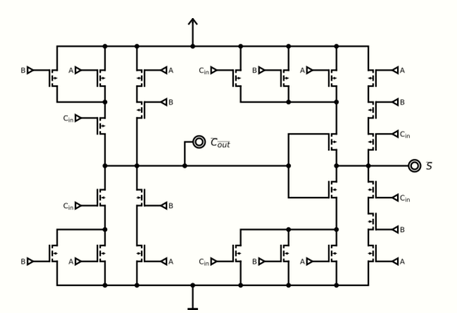Full adder with inverted outputs with single-transistor carry propagation delay in CMOS. https://handwiki.org/wiki/index.php?curid=2008858

### 1.3. Adders Supporting Multiple Bits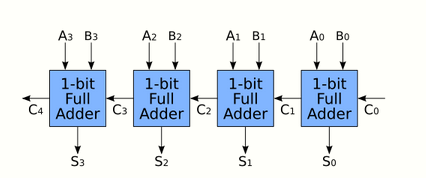4-bit adder with logical block diagram shown. https://handwiki.org/wiki/index.php?curid=2062779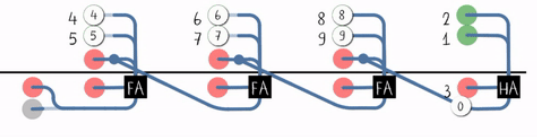$\displaystyle{ T_\text{CRA}(n) = T_\text{HA} + (n-1) \cdot T_\text{c} + T_\text{s} = T_\text{FA} + (n-1) \cdot T_c = 3 D + (n-1) \cdot 2 D = (2n+1) \cdot D. }$

A design with alternating carry polarities and optimized AND-OR-Invert gates can be about twice as fast.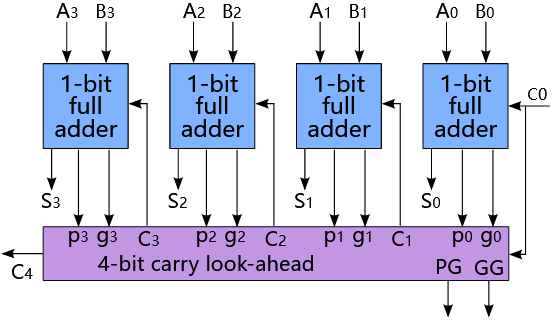To reduce the computation time, engineers devised faster ways to add two binary numbers by using carry-lookahead adders (CLA). They work by creating two signals (P and G) for each bit position, based on whether a carry is propagated through from a less significant bit position (at least one input is a 1), generated in that bit position (both inputs are 1), or killed in that bit position (both inputs are 0). In most cases, P is simply the sum output of a half adder and G is the carry output of the same adder. After P and G are generated, the carries for every bit position are created. Some advanced carry-lookahead architectures are the Manchester carry chain, Brent–Kung adder (BKA), and the Kogge–Stone adder (KSA).

Some other multi-bit adder architectures break the adder into blocks. It is possible to vary the length of these blocks based on the propagation delay of the circuits to optimize computation time. These block based adders include the carry-skip (or carry-bypass) adder which will determine P and G values for each block rather than each bit, and the carry-select adder which pre-generates the sum and carry values for either possible carry input (0 or 1) to the block, using multiplexers to select the appropriate result when the carry bit is known.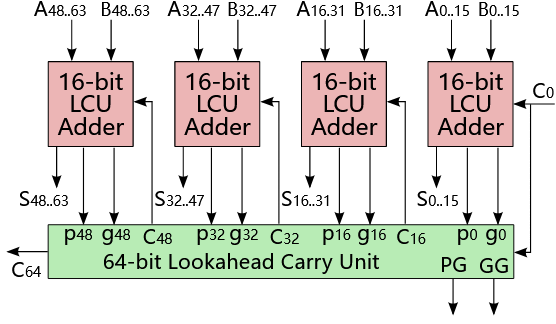By combining multiple carry-lookahead adders, even larger adders can be created. This can be used at multiple levels to make even larger adders. For example, the following adder is a 64-bit adder that uses four 16-bit CLAs with two levels of lookahead carry units.

If an adding circuit is to compute the sum of three or more numbers, it can be advantageous to not propagate the carry result. Instead, three-input adders are used, generating two results: a sum and a carry. The sum and the carry may be fed into two inputs of the subsequent 3-number adder without having to wait for propagation of a carry signal. After all stages of addition, however, a conventional adder (such as the ripple-carry or the lookahead) must be used to combine the final sum and carry results.

### 1.4. 3:2 Compressors

A full adder can be viewed as a 3:2 lossy compressor: it sums three one-bit inputs and returns the result as a single two-bit number; that is, it maps 8 input values to 4 output values. Thus, for example, a binary input of 101 results in an output of 1 + 0 + 1 = 10 (decimal number 2). The carry-out represents bit one of the result, while the sum represents bit zero. Likewise, a half adder can be used as a 2:2 lossy compressor, compressing four possible inputs into three possible outputs.

Such compressors can be used to speed up the summation of three or more addends. If the addends are exactly three, the layout is known as the carry-save adder. If the addends are four or more, more than one layer of compressors is necessary, and there are various possible designs for the circuit: the most common are Dadda and Wallace trees. This kind of circuit is most notably used in multipliers, which is why these circuits are also known as Dadda and Wallace multipliers.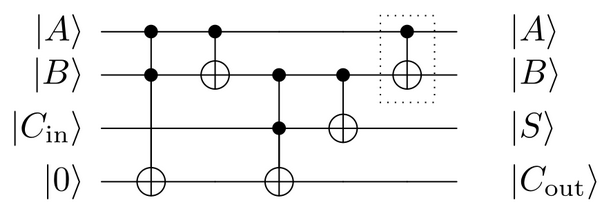Quantum full adder, using Toffoli and CNOT gates. The CNOT-gate that is surrounded by a dotted square in this picture can be omitted if uncomputation to restore the B output is not required. https://handwiki.org/wiki/index.php?curid=1300633

Using only the Toffoli and CNOT quantum logic gates, it is possible to produce quantum full- and half-adders. The same circuits can also be implemented in classical reversible computation, as both CNOT and Toffoli are also classical logic gates.

Since the quantum Fourier transform have a low circuit complexity, it can efficiently be used for adding numbers as well.

Just as in Binary adders, combining two input currents effectively adds those currents together. Within the constraints of the hardware, non-binary signals (i.e. with a base higher than 2) can be added together to calculate a sum. Also known as a "summing amplifier", this technique can be used to reduce the number of transistors in an addition circuit.

### References

1. Excel HSC Software Design and Development. Pascal Press. 2004. p. 180. ISBN 978-1-74125175-3. https://books.google.com/books?id=PZkDpS4m0fMC&pg=PA180.
2. Digital Logic and Computer Design. Prentice-Hall. 1979. pp. 119–123. ISBN 978-0-13-214510-7. https://archive.org/details/digitallogiccomp00mano/page/119.
4. Fischer, P.. "Einfache Schaltungsblöcke". Universität Heidelberg. https://sus.ziti.uni-heidelberg.de/Lehre/WS1617_DST/DST_Fischer_06_Einfache_Bloecke_FF.pptx.pdf. Retrieved 2021-09-05.
5. Design and Implementation of Carry Select Adder Using T-Spice. Anchor Academic Publishing. 2016. p. 22. ISBN 978-3-96067058-2. https://books.google.com/books?id=F_AWDQAAQBAJ&pg=PA22.
6. "Fast Ripple-Carry Adders in Standard-Cell CMOS VLSI". 20th IEEE Symposium on Computer Arithmetic. 2011. pp. 103–111. http://ieeexplore.ieee.org/Xplore/login.jsp?url=http%3A%2F%2Fieeexplore.ieee.org%2Fiel5%2F5991607%2F5992089%2F05992115.pdf%3Farnumber%3D5992115&authDecision=-203.
7. "A Regular Layout for Parallel Adders". IEEE Transactions on Computers C-31 (3): 260–264. March 1982. doi:10.1109/TC.1982.1675982. ISSN 0018-9340. http://www.dtic.mil/get-tr-doc/pdf?AD=ADA074455.
8. "A Parallel Algorithm for the Efficient Solution of a General Class of Recurrence Equations". IEEE Transactions on Computers C-22 (8): 786–793. August 1973. doi:10.1109/TC.1973.5009159.  https://dx.doi.org/10.1109%2FTC.1973.5009159
9. Reynders, Nele; Dehaene, Wim (2015). Ultra-Low-Voltage Design of Energy-Efficient Digital Circuits. Analog Circuits And Signal Processing (ACSP) (1 ed.). Cham, Switzerland: Springer International Publishing AG Switzerland. doi:10.1007/978-3-319-16136-5. ISBN 978-3-319-16135-8.  https://dx.doi.org/10.1007%2F978-3-319-16136-5
10. Feynman, Richard P. (1986). "Quantum mechanical computers". Foundations of Physics (Springer Science and Business Media LLC) 16 (6): 507–531. doi:10.1007/bf01886518. ISSN 0015-9018. Bibcode: 1986FoPh...16..507F.  https://dx.doi.org/10.1007%2Fbf01886518
11. "Code example: Quantum full adder". QuTech (Delft University of Technology (TU Delft) and the Netherlands Organisation for Applied Scientific Research (TNO)). https://www.quantum-inspire.com/kbase/full-adder/.
12. Dibyendu Chatterjee, Arijit Roy (2015). "A transmon-based quantum half-adder scheme". Progress of Theoretical and Experimental Physics 2015 (9). doi:10.1093/ptep/ptv122. Bibcode: 2015PTEP.2015i3A02C. https://paperity.org/p/73955611/a-transmon-based-quantum-half-adder-scheme.
13. Draper, Thomas G. (7 Aug 2000). "Addition on a Quantum Computer". arXiv:quant-ph/0008033. //arxiv.org/abs/quant-ph/0008033
14. Ruiz-Perez, Lidia; Juan Carlos, Garcia-Escartin (2 May 2017). "Quantum arithmetic with the quantum Fourier transform". Quantum Information Processing 16 (6): 152. doi:10.1007/s11128-017-1603-1. Bibcode: 2017QuIP...16..152R.  https://dx.doi.org/10.1007%2Fs11128-017-1603-1
15. "Summing Amplifier is an Op-amp Voltage Adder". 22 August 2013. https://www.electronics-tutorials.ws/opamp/opamp_4.html.
More
Information
Subjects:
Contributor MDPI registered users' name will be linked to their SciProfiles pages. To register with us, please refer to https://encyclopedia.pub/register :
View Times: 804
Entry Collection:
Revisions: 2 times (View History)
Update Date: 30 Nov 2022
1000/1000